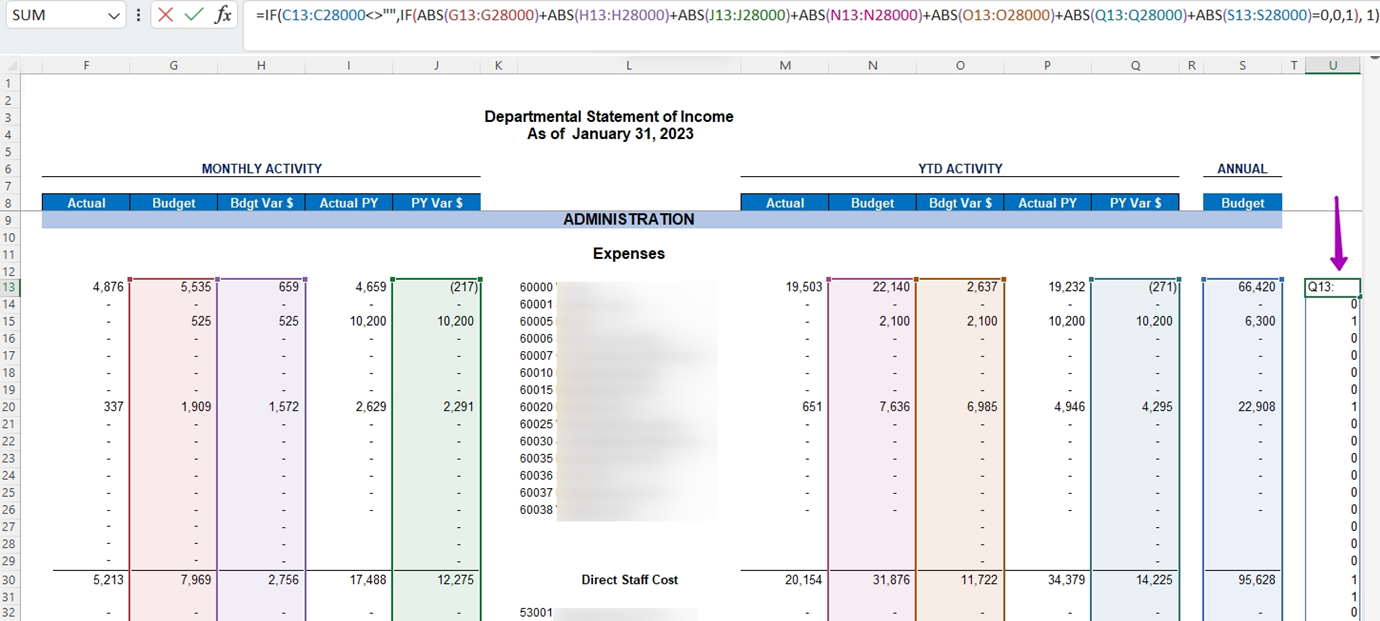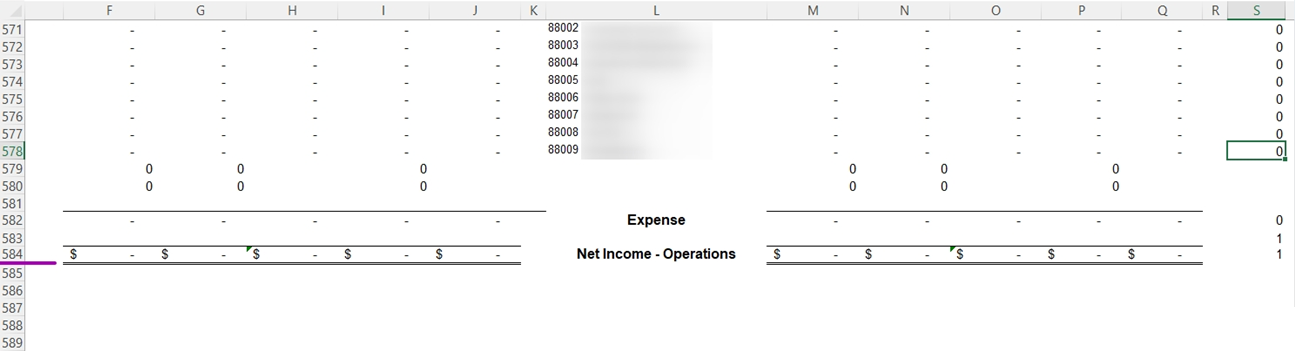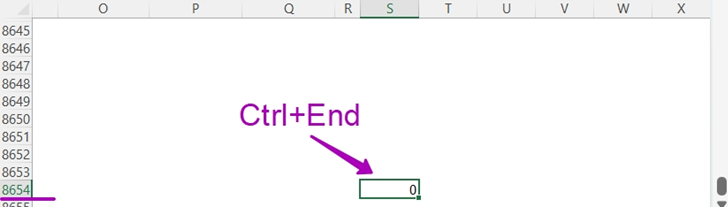Portal Categories Articles in this folder You may like to read

# Auto-Hide feature is very slow

Created by Harry Lewis, Modified on Tue, 05 Sep 2023 at 03:57 PM by Harry Lewis

# Applies to

Velixo Classic for Excel for Windows

# Overview

Sometimes you may experience an issue with the Velixo Auto-Hide feature where the workbook will freeze or is extremely slow.  Although this can be caused by many factors (including device in use, volatile functions, nested formulas, Excel version, etc.), there may be ways to mitigate the issues and improve performance.  This article addresses some of the possible causes.

## The situation that can cause this

• The workbook has many tabs (more than 20) with complex formulas (calculations) on every (or, at least, many) worksheets.

• One of the worksheets contains many rows (more than 20,000).

• Every worksheet has a specific column for use with the Auto-Hide feature and uses a formula in each row to calculate a Boolean value (0 – to hide the row, 1 – leave it).

• You are using Velixo Classic with Excel for Windows.

# Example

The example below illustrates the issue.  This workbook has 30 worksheets, and one worksheet has over 27,000 rows.  There is a formula in every row of column U to calculate the value for auto-hide (either a 0 or 1).

That formula looks like this:

`=IF(OR(F8953<>0,G8953<>0,I8953<>0,M8953<>0,N8953<>0,P8953<>0)=TRUE,1,0)`Once an Auto-Hide range is selected in column U and the option Hide Zero Rows -> Auto Hide / Unhide Rows in Selected Range is selected, it took about 5 minutes to be hidden.  Obviously, this is not the desired outcome.

## Method 1 - Using dynamic ranges for setting an Auto-Hide range

One of the most important ways to improve performance is replacing by-row formulas with dynamic ranges.  So, you would have only one formula with a dynamic range instead of a formula in every row.

`=IF(C13:C50000<>"",IF(ABS(G13:G50000)+ABS(H13:H50000)+ABS(J13:J50000)+ABS(N13:N50000)+ABS(O13:O50000)+ABS(Q13:Q50000)+ABS(S13:S50000)=0,0,1), 1)`Excel is able to calculate that one formula much faster than it can the over 27,000 formulas (thus, improving the performance of the Auto-Hide feature).

## Method 2 - Checking the data end by using Ctrl+End

Another possible way to optimize the performance is to check whether the real end of data matches with Excel’s Ctrl+End feature.  Pressing Ctrl-End will select the last cell in your worksheet.  If there are more cells than are needed, then those empty rows should be deleted.

For example, our data ends at row 584:But by using the Ctrl-End feature, we see that Excel considers the end of the worksheet to be in row 8654:Be deleting the extra rows (and then saving the workbook, restarting Excel, and re-opening the workbook), Excel may now be able to apply the Auto-Hide feature much faster.

## Method 3 - Using other functions

Another way to achieve faster calculations is to use some unified Velixo functions.  So, instead of using a few different functions, you can use just one function.

For example, use either:

• ACCOUNTSANDSUBACCOUNTSWITHHISTORY (if the data you want to hide zeroes in is GL data) or
• EXPANDPROJECTHISTORY (for Project Module data)

These functions can return multiple columns of ERP data and can automatically exclude "all zero" data (so that you do not need to use one of the Velixo Hide features).